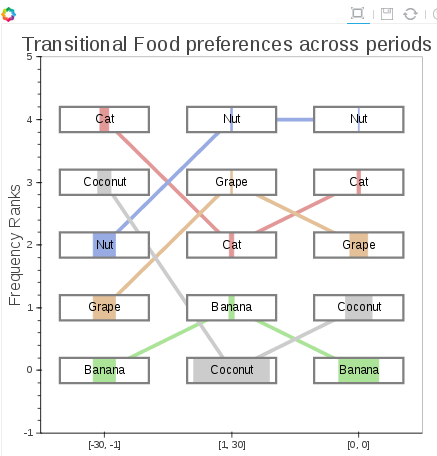# offsets for rect

I have been cleaning up my example as found in:

https://github.com/bokeh/bokeh/issues/1564

I realized there are some enhancements that could be made to make it easier to interpret.

so I added several more rect’s to get the attached visual output.The issue is, I cannot seem to find anyway to set an offset for the colored rectangles.

I looked into:

https://github.com/bokeh/bokeh/blob/master/bokeh/_glyph_functions.py

On that page I see it is specifying line_properties and fill_properties:

"

In addition the the parameters specific to this glyph,

:ref:`userguide_objects_line_properties` and :ref:`userguide_objects_fill_properties`

are also accepted as keyword parameters.

"

The filled areas are the % of the column that is taken up by that food preference, but I think it would be quite preferable to have them left aligned within the gray box. I believe I could work that out with an offset.

Here is the full example:

import bokeh.plotting as bk

import pandas as pd

#output goes to the notebook

bk.output_notebook()

#prepare the data

df = pd.DataFrame([

[0, ‘Banana’, “[-30, -1]”, 0.25],

[1, ‘Grape’, “[-30, -1]”, 0.25],

[2, ‘Nut’, “[-30, -1]”, 0.25],

[3, ‘Coconut’, “[-30, -1]”, 0.15],

[4, ‘Cat’, “[-30, -1]”, 0.10],

[0, ‘Banana’, “[0, 0]”, 0.45],

[1, ‘Coconut’, “[0, 0]”, 0.30],

[2, ‘Grape’, “[0, 0]”, 0.20],

[3, ‘Cat’, “[0, 0]”, 0.04],

[4, ‘Nut’, “[0, 0]”, 0.01],

[0, ‘Coconut’, “[1, 30]”, 0.85],

[1, ‘Banana’, “[1, 30]”, 0.06],

[2, ‘Cat’, “[1, 30]”, 0.05],

[3, ‘Grape’, “[1, 30]”, 0.02],

[4, ‘Nut’, “[1, 30]”, 0.02],

],

columns=[‘rankof’,‘typeof’,‘periodof’, ‘perof’]

)

x_axis_labels = list(set(df.periodof))

color_list = [’#E39898’, ‘#E3C098’, ‘#ABE398’, ‘#98ABE3’, ‘#CCCCCC’ ]

#color_list = [‘red’,‘orange’,‘green’,‘blue’,‘black’]

list_of_levels = list(set(df.typeof))

shortened_text = [x[:14] for x in df.typeof]

colors = [color_list for x in

[list_of_levels.index(x) for x in df.typeof]

]

#build out segment data

segments =

for level in list_of_levels:

for i in range(len(x_axis_labels)-1):

segments.append([

(x_axis_labels[i], list(df.rankof[(df.typeof == level) & (df.periodof == x_axis_labels[i])])),

(x_axis_labels[i+1], list(df.rankof[(df.typeof == level) & (df.periodof == x_axis_labels[i+1])])),

color_list[list_of_levels.index(level)],

level

])

#create a new figure

p = bk.figure(

title=“Transitional Food preferences across periods”,

y_range=[-1,5],

x_range=x_axis_labels

)

#create the plot

p.segment(

[x for x in segments],

[x for x in segments],

[x for x in segments],

[x for x in segments],

color=[x for x in segments],

line_alpha=1,

fill_alpha=1,

line_width=5

)

B_WIDTH = .7

B_HEIGHT = .4

#setup white rect to obscure the line in background

p.rect(df.periodof, df.rankof, B_WIDTH, B_HEIGHT, color="#FFFFFF",fill_alpha=1)

#setup color feels according to the percent

p.rect(df.periodof, df.rankof, B_WIDTH*df.perof, B_HEIGHT, color=colors,fill_alpha=1)

#setup outline to bound the effictive region.

p.rect(df.periodof, df.rankof, B_WIDTH, B_HEIGHT, color=None, fill_alpha=1, line_color="#808080", line_width=3)

#p.rect(df.periodof, df.rankof, B_WIDTH, B_HEIGHT, color=colors,fill_alpha=1, line_color="#808080")

p.text(df.periodof, df.rankof, shortened_text, 0,

text_align=“center”, text_baseline=“middle”)

# nope p.line(df.periodof,df.rankof)

#customize axes

xa, ya = p.axis

xa.axis_label = ‘Day_sep ranges’

ya.axis_label = ‘Frequency Ranks’

p.xgrid.grid_line_color = None

p.ygrid.grid_line_color = None

#show the plots

bk.show§